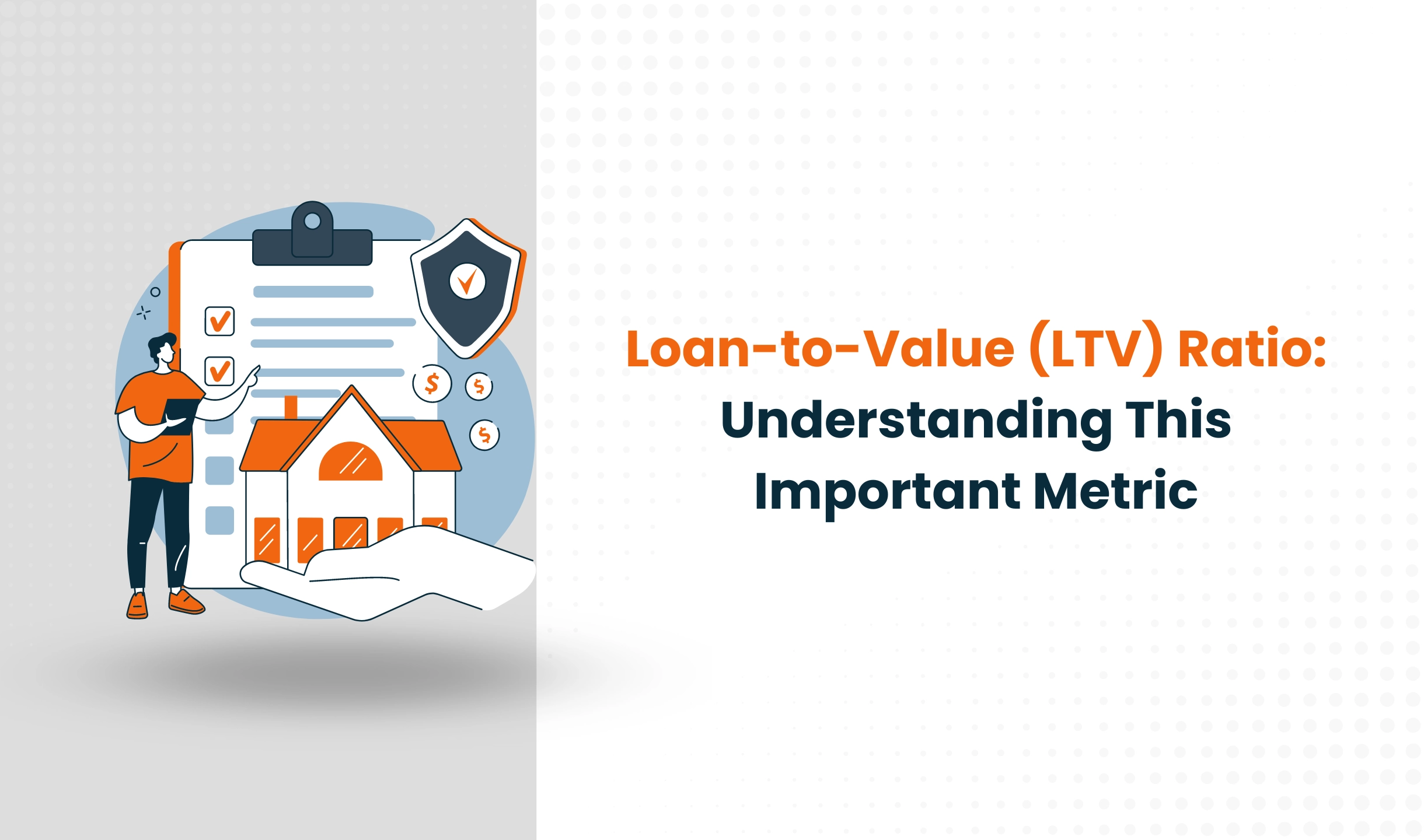Startup
December 29, 2022

# Loan-to-Value (LTV) Ratio: Understanding This Important MetricThe Restaurant Business An Accounting Guide

If you're in the process of applying for a mortgage or refinancing your existing home loan, you may have come across the term "loan-to-value (LTV) ratio." But what exactly is this ratio, and how does it impact your loan application? In this blog post, we'll explore the LTV ratio and how to calculate it, as well as provide some examples to help you better understand this important concept.

## What is the Loan-to-Value (LTV) Ratio?

The LTV ratio is a measure of the size of your loan compared to the value of the property you're using as collateral. It's expressed as a percentage and is calculated by dividing the loan amount by the property's value.

For example, if you're applying for a \$200,000 mortgage on a property with a value of \$250,000, your LTV ratio would be 80% (200,000 / 250,000 = .8). This means that the loan represents 80% of the property's value.

How is the LTV Ratio Used?

Lenders use the LTV ratio to determine the level of risk involved in lending you money. A higher LTV ratio means that the loan represents a larger percentage of the property's value, which means that there is more risk for the lender. As a result, lenders may require a higher down payment or charge a higher interest rate for loans with a higher LTV ratio.

On the other hand, a lower LTV ratio means that the loan represents a smaller percentage of the property's value, which means that there is less risk for the lender. In this case, lenders may be more likely to approve the loan and offer a lower interest rate.

How to Calculate the LTV Ratio

### Calculating the LTV ratio is relatively simple. To do so, follow these steps:

1. Determine the value of the property: This is typically done by an appraiser, who will assess the value of the property based on factors such as location, size, age, and condition.
2. Determine the loan amount: This is the amount of money that you are borrowing from the lender.
3. Divide the loan amount by the property value: For example, if you're applying for a \$200,000 mortgage on a property with a value of \$250,000, your LTV ratio would be 80% (200,000 / 250,000 = .8).

It's important to note that the LTV ratio is only one factor that lenders consider when evaluating a loan application. Other factors may include your credit score, income, debt-to-income ratio, and employment history.

### Example of the LTV Ratio in Action

To further illustrate how the LTV ratio works, let's consider the following example:

Alice is looking to purchase a home for \$400,000 and has saved up \$80,000 for a down payment. This means that she will need to borrow \$320,000 to cover the remaining cost of the home. Based on an appraisal, the lender determines that the property is worth \$400,000.

In this case, Alice's LTV ratio would be 80% (320,000 / 400,000 = .8). This is considered a relatively high LTV ratio, as the loan represents a significant portion of the property's value. As a result, the lender may require a higher down payment or charge a higher interest rate to compensate for the higher level of risk.

On the other hand, if Alice had saved up \$120,000 for a down payment, her LTV ratio would be 60% (320,000 / 400,000 = .6). This is considered a lower LTV ratio, as the loan represents a smaller percentage of the property's value. As a result, the lender may be more likely to approve the loan and offer a lower interest rate.

It's important to note that the LTV ratio is only one factor that lenders consider when evaluating a loan application. Other factors may include your credit score, income, debt-to-income ratio, and employment history.

Understanding the LTV ratio can be helpful when you're shopping for a mortgage or refinancing your existing home loan. By having a good understanding of your LTV ratio, you can negotiate with lenders to get the best possible terms on your loan.

### Conclusion

The LTV ratio is an important metric that lenders use to assess the risk involved in lending you money. It's calculated by dividing the loan amount by the value of the property you're using as collateral. A lower LTV ratio typically indicates a lower level of risk for the lender, which may result in a lower interest rate or more favorable loan terms. Understanding the LTV ratio can help you negotiate the best possible terms on your mortgage or refinancing loan.